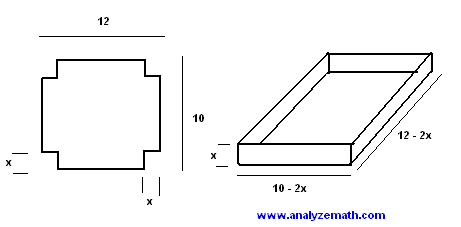# Maximize Volume of a Box Optimization Problem

How to maximize the volume of a box using the first derivative of the volume. Volume optimization problem with solution.

## Problem

A sheet of metal 12 inches by 10 inches is to be used to make a open box. Squares of equal sides x are cut out of each corner then the sides are folded to make the box. Find the value of x that makes the volume maximum.Solution to Problem 1:

• We first use the formula of the volume of a rectangular box.
V = L * W * H
• The box to be made has the following dimensions:
L = 12 - x
W = 10 - 2x
H = x
• We now write the volume of the box to ba made as follows:
V(x) = x (12 - 2x) (10 - 2x) = 4x (6 - x) (5 - x)
= 4x (x 2 -11 x + 30)
• We now determine the domain of function V(x). All dimemsions of the box must be positive or zero, hence the conditions
x > = 0 and 6 - x > = 0 and 5 - x > = 0
• Solve the above inequalities and find the intersection, hence the domain of function V(x)
0 < = x < = 5
• Let us now find the first derivative of V(x) using its last expression.
dV / dx = 4 [ (x 2 -11 x + 3) + x (2x - 11) ]
= 3 x 2 -22 x + 30
• Let us now find all values of x that makes dV / dx = 0 by solving the quadratic equation
3 x 2 -22 x + 30 = 0
• Two values make dV / dx = 0: x = 5.52 and x = 1.81, rounded to one decimal place. x = 5.52 is outside the domain and is therefore rejected. Let us now examine the values of V(x) at x = 1.81 and the endpoints of the domain.
V(0) = 0 , v(5) = 0 and V(1.81) = 96.77 (rounded to two decimal places)
• So V(x) is maximum for x = 1.81 inches. The graph of function V(x) is shown below and we can clearly see that there is a maximum very close to 1.8.More references on calculus problems